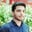Related Tags

java
math
communitycreator

# How to use Math atan2(double y, double x) in JavaSalman Yousaf

The atan2() function in Java accepts two argument values, x and y. It computes a radian angle Θ in the euclidean plane between the positive x-axis and the ray to the point.

What is Θ?

### Basic formulas

The formulas below can be used to calculate arctan(y/x).

1. x = rcosθ)
2. y = rsin(θ)
3. θ = arctan(y/x) or atan2()

### Syntax

double atan2(double y, double x)


### Parameters

It accepts two arguments of the primitive double type.

• x: value on the x-axis named abscissa.
• y: value on the y-axis named ordinate.

### Return value

The return value is the Θ component of the point (r, Θ) in polar coordinates corresponding to the point (x, y) in the Cartesian plane.

• (x, y) ≠ (0, 0)

### Special cases

• If the argument passed to it is NaN, the result will be NaN.

• If both x and y arguments are positive infinity, the result will be nearest to π/4 of the primitive double type.

• If both argument values are negative infinity, the result will be nearest to -3*$\frac{pi}{4}$ of the primitive double type.

• If the $1^{st}$ argument is positive infinity and the $2^{nd}$ argument is negative infinity, the result will be 3*$\frac{pi}{4}$ of the primitive double type.

• If the $1^{st}$ argument is negative infinity and the $2^{nd}$ argument is positive infinity, the result will be -$\frac{pi}{4}$ of the primitive double type.

### Code

In the code snippet below:

• (0, 0): if the value of x= 0, y= 0 ,the angle will be 0.

• (10, 3): if the value of x= 10, y= 3, the angle will be 1.2793395323170296.

Execute the code below and check the output.

class EdPresso {
public static void main(String args[]) {
double x= 0, y= 0;
System.out.println( "Radian angle Θ in euclidean plane(" + x + "," + y + ")= "  + Math.atan2(x,y));

x= 10; y= 3;
System.out.println( "Radian angle Θ in euclidean plane(" + x + "," + y + ")= "  + Math.atan2(x,y));
}
}

RELATED TAGS

java
math
communitycreator

CONTRIBUTORSalman Yousaf
RELATED COURSES

View all Courses

Keep Exploring

Learn in-demand tech skills in half the time